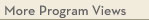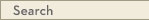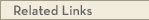# University Catalogs

Morris campus

This is archival data. This system was retired as of August 21, 2023 and the information on this page has not been updated since then. For current information, visit catalogs.umn.edu.Morris Campus

# Mathematics Minor

Division of Science & Mathematics - Adm
Division of Science and Mathematics
• Program Type: Undergraduate minor related to major
• Requirements for this program are current for Spring 2024
• Required credits in this minor: 26
The mission of the discipline is to advance knowledge of mathematics by teaching mathematics and its processes, by research in mathematics and mathematical pedagogy, and by dissemination of this knowledge to students and the community we serve.
Program Delivery
This program is available:
• via classroom (the majority of instruction is face-to-face)
Minor Requirements
Courses may not be taken S-N unless offered S-N only. Up to 5 credits of coursework with a grade of D or D+ may be used to meet the minor requirements if offset by an equivalent number of credits of B- or above in courses at or above the 2XXX level. The GPA in these courses must be at least 2.00.
Required Courses
MATH 1101 - Calculus I [M/SR] (5.0 cr)
MATH 1102 - Calculus II [M/SR] (5.0 cr)
MATH 3111 - Linear Algebra (4.0 cr)
Elective Courses
Take 12 or more credit(s) from the following:
· MATH 2101 - Calculus III [M/SR] (4.0 cr)
· MATH 2202 - Mathematical Perspectives [M/SR] (4.0 cr)
· MATH 2211 - History of Mathematics (4.0 cr)
· MATH 2212 - Introduction to Knot Theory [M/SR] (4.0 cr)
· MATH 2401W - Differential Equations [M/SR] (4.0 cr)
· MATH 2452 - Introduction to Mathematical Modeling (4.0 cr)
· MATH 2501 - Probability and Stochastic Processes [M/SR] (4.0 cr)
· MATH 3211 - Geometry [M/SR] (4.0 cr)
· MATH 3221 - Real Analysis I (4.0 cr)
· MATH 3222 - Complex Analysis (2.0 cr)
· MATH 3231 - Abstract Algebra I (4.0 cr)
· MATH 3401 - Operations Research (4.0 cr)
· MATH 3411 - Discrete and Combinatorial Mathematics (4.0 cr)
· MATH 4211 - Real Analysis II (2.0 cr)
· MATH 4221 - Topology (2.0 cr)
· MATH 4231 - Abstract Algebra II (2.0 cr)
· MATH 4241 - Number Theory (2.0 cr)
· MATH 4252 - Differential Geometry (2.0 cr)
· MATH 4253 - Combinatorics (2.0 cr)
· MATH 4401 - Numerical Methods with Applications in Mathematical Modeling (4.0 cr)
· One of the courses below can be chosen to fulfill 4 elective credits for the math minor
· STAT 2601 - Statistical Methods [M/SR] (4.0 cr)
or STAT 2611 - Mathematical Statistics [M/SR] (4.0 cr)View college catalog(s): · Division of Science and Mathematics View sample plan(s): · sample plan 1 · sample plan 2 · sample plan 3 View checkpoint chart: · Mathematics Minor View PDF Version:Search Programs Search University CatalogsOne Stop for tuition, course registration, financial aid, academic calendars, and moreMATH 1101 - Calculus I (M/SR)
 Credits: 5.0 [max 5.0] Typically offered: Every Fall & Spring
Limits and continuity; the concepts, properties, and some techniques of differentiation, antidifferentiation, and definite integration and their connection by the Fundamental Theorem. Partial differentiation. Some applications. Students learn the basics of a computer algebra system. prereq: 1012, 1013 or placement
MATH 1102 - Calculus II (M/SR)
 Credits: 5.0 [max 5.0] Typically offered: Every Fall & Spring
Techniques of integration. Further applications involving mathematical modeling and solution of simple differential equations. Taylor's Theorem. Limits of sequences. Use and theory of convergence of power series. Students use a computer algebra system. prereq: 1101
MATH 3111 - Linear Algebra
 Credits: 4.0 [max 4.0] Typically offered: Every Fall & Spring
Math majors are highly encouraged to take this course in their second year. Matrix algebra, systems of linear equations, finite dimensional vector spaces, linear transformations, determinants, inner-product spaces, characteristic values and polynomials, eigenspaces, minimal polynomials, diagonalization of matrices, related topics; applications. [Note: no credit for students who have received cr for Math 2111] prereq: 1102 or CSci 1302 or instr consent
MATH 2101 - Calculus III (M/SR)
 Credits: 4.0 [max 4.0] Typically offered: Every Fall & Spring
Multivariable and vector calculus. Three-dimensional analytic geometry; partial differentiation; multiple integration; gradient, divergence, and curl; line and surface integrals; divergence theorem; Green and Stokes theorems; applications. prereq: 1102 or instr consent
MATH 2202 - Mathematical Perspectives (M/SR)
 Credits: 4.0 [max 4.0] Typically offered: Every Spring
Introduction to the methodology and subject matter of modern mathematics. Logic, sets, functions, relations, cardinality, and induction. Introductory number theory. Roots of complex polynomials. Other selected topics. prereq: 1101
MATH 2211 - History of Mathematics
 Credits: 4.0 [max 4.0] Prerequisites: 1101 or higher or # Typically offered: Fall Even Year
Historical development of various areas in mathematics and important figures in mathematics from ancient to modern times. prereq: 1101 or higher or instr consent
MATH 2212 - Introduction to Knot Theory (M/SR)
 Credits: 4.0 [max 4.0] Typically offered: Periodic Fall
Introduction to the mathematical study of knots. Presentation, tabulation, and invariants of knots. Additional selected topics from low-dimensional topology. prereq: 1101 or higher or instr consent
MATH 2401W - Differential Equations (M/SR)
 Credits: 4.0 [max 4.0] Typically offered: Every Fall
First-order and second-order differential equations with methods of solution and applications, Laplace transforms, systems of equations, series solutions, existence and uniqueness theorems, the qualitative theory of differential equations. prereq: 1102 or instr consent
MATH 2452 - Introduction to Mathematical Modeling
 Credits: 4.0 [max 4.0] Typically offered: Periodic Fall & Spring
Mathematical topics include, but are not limited to, differential and difference equations, discrete and continuous dynamical systems, predator-prey models, discrete and continuous optimization models, probabilistic models, stochastic and Poisson processes, and queuing models. Applications are drawn from different areas in the sciences and social sciences. [Note: no credit for students who have received cr for Math 4452] prereq: 1101 or 1021 or instr consent
MATH 2501 - Probability and Stochastic Processes (M/SR)
 Credits: 4.0 [max 4.0] Course Equivalencies: Math 2501/Stat 2501 Typically offered: Every Fall
Same as Stat 2501. Probability theory; set theory, axiomatic foundations, conditional probability and independence, Bayes' rule, random variables. Transformations and expectations; expected values, moments, and moment generating functions. Common families of distributions; discrete and continuous distributions. Multiple random variables; joint and marginal distributions, conditional distributions and independence, covariance and correlation, multivariate distributions. Properties of random sample and central limit theorem. Markov chains, Poisson processes, birth and death processes, and queuing theory. prereq: 1101 or instr consent
MATH 3211 - Geometry (M/SR)
 Credits: 4.0 [max 4.0] Prerequisites: 1102 or higher or # Typically offered: Fall Odd Year
Synthetic approach to Euclidean and non-Euclidean geometries. Selected topics from affine, hyperbolic, spherical, projective geometries. Possible comparisons of analytic and synthetic approaches. May include other related topics or use of computer software for geometry. prereq: 1102 or higher or instr consent
MATH 3221 - Real Analysis I
 Credits: 4.0 [max 4.0] Prerequisites: 1102, 2202 or # Typically offered: Every Fall
Introduction to real analysis. The main topics of single-variable calculus-convergence, continuity, differentiation, and series as they are applied and extended in advanced settings with emphasis on precise statements and rigorous proofs. Structure of the real numbers, open and closed sets. Integration, metric spaces, and other topics and applications as time allows. prereq: 1102, 2202 or instr consent
MATH 3222 - Complex Analysis
 Credits: 2.0 [max 2.0] Typically offered: Periodic Fall & Spring
Differentiable and analytic functions of a complex variable. Contour integral theorems. Laurent expansions. Other topics optional. prereq: 2101 or 3221 or instr consent
MATH 3231 - Abstract Algebra I
 Credits: 4.0 [max 4.0] Typically offered: Every Spring
Systematic study of groups and rings, making use of linear algebra. Groups as codifying symmetry throughout mathematics and its applications. The Euclidean algorithm and its consequences, both for integers and polynomials. Other selected topics and applications. prereq: 3111, 2202 or instr consent
MATH 3401 - Operations Research
 Credits: 4.0 [max 4.0] Typically offered: Periodic Fall & Spring
Topics include, but not limited to, linear and integer linear programming formulations, sensitivity analysis and duality, network models and applications. prereq: 1101 or higher or instr consent
MATH 3411 - Discrete and Combinatorial Mathematics
 Credits: 4.0 [max 4.0] Prerequisites: 1102 or higher or # Typically offered: Every Fall
Propositional logic; equivalence relations; recurrence equations; structures and properties of undirected and directed graphs; applications of the aforementioned topics. prereq: 1102 or higher or instr consent
MATH 4211 - Real Analysis II
 Credits: 2.0 [max 2.0] Prerequisites: 3221 or # Typically offered: Periodic Fall & Spring
Differentiation of functions of several variables. The extension of integration to other forms of integrals. Introduction to measure theory. Other optional topics. prereq: 3221 or instr consent
MATH 4221 - Topology
 Credits: 2.0 [max 2.0] Typically offered: Periodic Fall & Spring
Selected topics from point set topology and/or algebraic topology. prereq: 2202 or instr consent
MATH 4231 - Abstract Algebra II
 Credits: 2.0 [max 2.0] Typically offered: Periodic Fall & Spring
Selected topics from the theory of finite groups, Galois theory of fields, and/or the theory of rings. prereq: 3231 or instr consent
MATH 4241 - Number Theory
 Credits: 2.0 [max 2.0] Typically offered: Periodic Fall & Spring
Selected topics from modular congruences, theory of primes, classical Diophantine equations, and the connections with algebraic curves. prereq: 2202 or instr consent
MATH 4252 - Differential Geometry
 Credits: 2.0 [max 2.0] Typically offered: Periodic Fall & Spring
Geometry of curves and surfaces. Frames, curvature, torsion, orientation, differential forms, topological properties of surfaces. The notion of differentiable manifold. Selected applications. prereq: instr consent
MATH 4253 - Combinatorics
 Credits: 2.0 [max 2.0] Typically offered: Periodic Fall & Spring
Selected topics from graph theory, the theory of ordered sets, and/or enumerative combinatorics. prereq: instr consent
MATH 4401 - Numerical Methods with Applications in Mathematical Modeling
 Credits: 4.0 [max 4.0] Typically offered: Periodic Fall & Spring
Finite differences; interpolation; numerical integration; numerical solutions of differential, algebraic, and transcendental equations; continuous mathematical models. prereq: 2401 or instr consent
STAT 2601 - Statistical Methods (M/SR)
 Credits: 4.0 [max 4.0] Typically offered: Every Fall
Descriptive statistics, elementary probability theory; laws of probability, random variables, discrete and continuous probability models, functions of random variables, mathematical expectation. Statistical inference; point estimation, interval estimation, tests of hypotheses. Other statistical methods; linear regression and correlation, ANOVA, nonparametric statistics, statistical quality control, use of statistical computer packages. prereq: Math 1101 or Math 1021
STAT 2611 - Mathematical Statistics (M/SR)
 Credits: 4.0 [max 4.0] Typically offered: Every Spring
Introduction to probability theory. Principles of data reduction; sufficiency principle. Point estimation; methods of finding and evaluating estimators. Hypothesis testing; methods of finding and evaluating tests. Interval estimation; methods of finding and evaluating interval estimators. Linear regression and ANOVA. prereq: Math 1101